PointGeometry

Resumen

A PointGeometry object is a shape that has neither length nor area at a given scale.

Debate

En muchos flujos de trabajo de geoprocesamiento, puede que necesite ejecutar una operación concreta utilizando información de coordenadas y geometría, pero que no necesariamente desee pasar por el proceso de crear una nueva clase de entidad (temporal), llenar la clase de entidad con cursores, utilizar la clase de entidad y, a continuación, eliminar la clase de entidad temporal. En su lugar, puede utilizar objetos de geometría tanto para la entrada como para la salida con el fin de facilitar el geoprocesamiento. Los objetos de geometría se pueden crear desde cero usando clases Geometry, Multipoint, PointGeometry, Polygon o Polyline.

During the creation of a geometry object, a simplification process is performed to make the geometry topologically consistent according to its geometry type. For instance, it rectifies polygons that may be self-intersecting, or contain incorrect ring orientations. Coordinates may move within the resolution of the geometry's spatial reference.

The following numeric operators can be used in place of geometry methods:

Geometry methodNumeric operator

intersect

+

union

|

difference

-

symmetricDifference

^

For example, to union two geometry objects, g3 = g1 | g2 is equivalent to g3 = g1.union(g2).

The + operator will use the geometry type (dimension) of the first geometry to determine the geometry type of the output.

Sintaxis

PointGeometry  (inputs, {spatial_reference}, {has_z}, {has_m})
 Parámetro Explicación Tipo de datos inputs The Point used to create the object. Point spatial_reference The spatial reference of the new geometry.(El valor predeterminado es None) SpatialReference has_z The Z state: True for geometry if Z is enabled and False if it is not. (El valor predeterminado es False) Boolean has_m The M state: True for geometry if M is enabled and False if it is not.(El valor predeterminado es False) Boolean

JSON
(Sólo lectura)

Returns an Esri JSON representation of the geometry as a string.

Sugerencia:

The returned string can be converted to a dictionary using the json module's loads function.

String
WKB
(Sólo lectura)

Returns the well-known binary (WKB) representation for OGC geometry. It provides a portable representation of a geometry value as a contiguous stream of bytes.

Bytearray
WKT
(Sólo lectura)

Returns the well-known text (WKT) representation for OGC geometry. It provides a portable representation of a geometry value as a text string.

Any true curves in the geometry will be densified into approximate curves in the WKT string.

String
area
(Sólo lectura)

The area of a polygon feature. It is zero for all other feature types.

Double
centroid
(Sólo lectura)

The true centroid if it is within or on the feature; otherwise, the label point is returned.

Point
extent
(Lectura y escritura)

The extent of the geometry.

Extent
firstPoint
(Sólo lectura)

The first coordinate point of the geometry.

Point
hasCurves
(Sólo lectura)

Returns True if the geometry has a curve.

Boolean
hullRectangle
(Sólo lectura)

A space-delimited string of the coordinate pairs of the convex hull rectangle.

String
isMultipart
(Sólo lectura)

Returns True if the number of parts for this geometry is more than one.

Boolean
labelPoint
(Sólo lectura)

The point at which the label is located. The labelPoint is always located within or on a feature.

Point
lastPoint
(Sólo lectura)

The last coordinate of the feature.

Point
length
(Sólo lectura)

The length of the linear feature. The calculation uses 2D Cartesian mathematics.

For point and multipoint geometry, the length will be zero. For polygon geometry, the length will be the 2D length of the boundary.

Double
length3D
(Sólo lectura)

The 3D length of the linear feature. The calculation uses 3D Cartesian mathematics.

For point and multipoint geometry, the length will be zero. For polygon geometry, the length will be the 3D length of the boundary.

Precaución:

The operation does not perform unit conversion between the horizontal and the vertical coordinate systems. The x, y, and z coordinates are assumed to be in the same linear unit.

Precaución:

This property is only available for projected data.

Double
partCount
(Sólo lectura)

The number of geometry parts for the feature.

Integer
pointCount
(Sólo lectura)

The total number of points for the feature.

Integer
spatialReference
(Sólo lectura)

The spatial reference of the geometry.

SpatialReference
trueCentroid
(Sólo lectura)

The center of gravity for a feature.

Point
type
(Sólo lectura)

The geometry type: polygon, polyline, point, multipoint, multipatch, dimension, or annotation.

String

Descripción general del método

 Método Explicación angleAndDistanceTo (other, {method}) Returns a tuple of angle and distance to a point or polygon. boundary () Constructs the boundary of the geometry.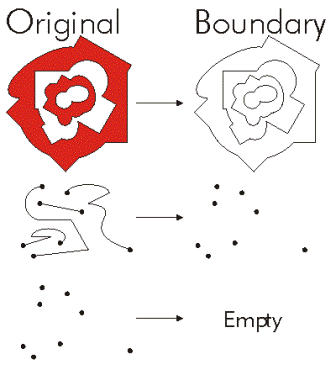buffer (distance) Constructs a polygon at a specified distance from the geometry.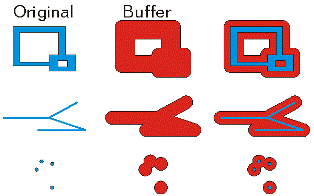clip (envelope) Constructs the intersection of the geometry and the specified extent.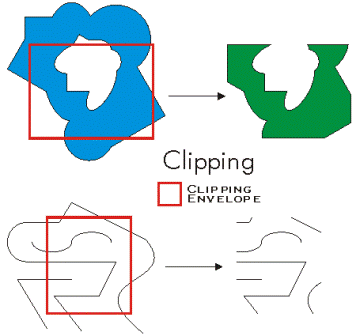contains (second_geometry, {relation}) Indicates if the base geometry contains the comparison geometry. contains is the opposite of within. Only True relationships are shown in this illustration.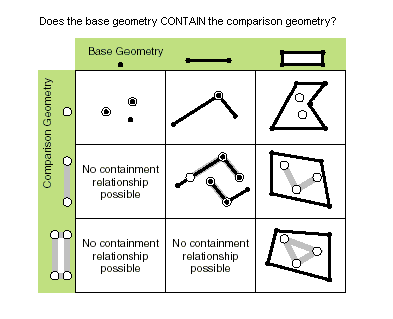convexHull () Constructs the geometry that is the minimal bounding polygon such that all outer angles are convex.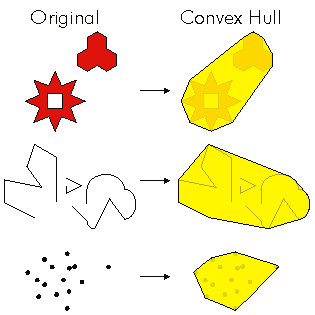crosses (second_geometry) Indicates if the two geometries intersect in a geometry of a lesser shape type. Two polylines cross if they share only points in common, at least one of which is not an endpoint. A polyline and an polygon cross if they share a polyline or a point (for vertical line) in common on the interior of the polygon which is not equivalent to the entire polyline. Only True relationships are shown in this illustration.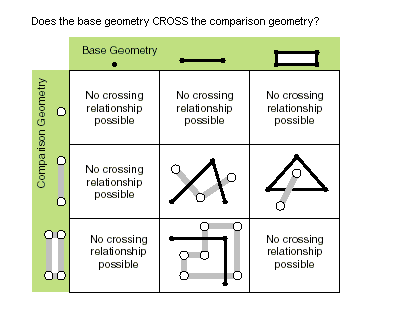difference (other) Constructs the geometry that is composed only of the region unique to the base geometry but not part of the other geometry. The following illustration shows the results when the red polygon is the source geometry.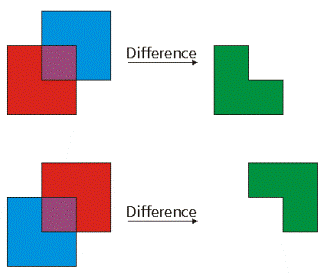disjoint (second_geometry) Indicates if the base and comparison geometries share no points in common. Two geometries intersect if disjoint returns False. Only True relationships are shown in this illustration.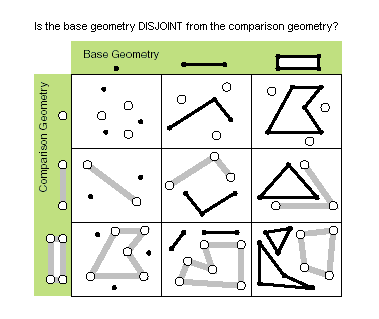distanceTo (other) Returns the minimum distance between two geometries. The distance is in the units of the geometry's spatial reference. If the geometries intersect, the minimum distance is 0.Both geometries must have the same projection. equals (second_geometry) Indicates if the base and comparison geometries are of the same shape type and define the same set of points in the plane. This is a 2D comparison only; M and Z values are ignored.Only True relationships are shown in this illustration.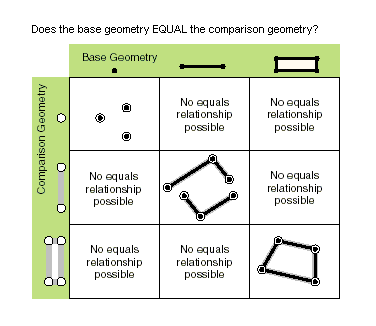getGeohash (precision) Converts a PointGeometry in geographic coordinate system coordinates of latitude and longitude to a geohash string that is accurate to an arbitrary precision within a bounding box in the geohash grid. getPart ({index}) Returns an Array object of Point objects for a particular part of the geometry if an index is specified. If an index is not specified, an Array object containing an Array of Point objects for each geometry part is returned. The getPart method is equivalent to indexing an object; that is, obj.getPart(0) is equivalent to obj. intersect (other, dimension) Constructs a geometry that is the geometric intersection of the two input geometries. Different dimension values can be used to create different shape types. The intersection of two geometries of the same shape type is a geometry containing only the regions of overlap between the original geometries.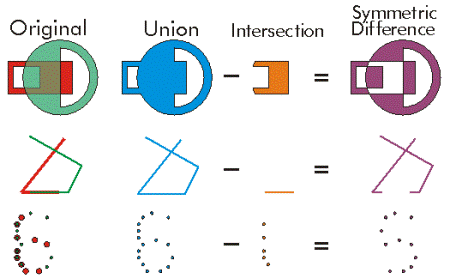For faster results, test if the two geometries are disjoint before calling intersect. overlaps (second_geometry) Indicates if the intersection of the two geometries has the same shape type as one of the input geometries and is not equivalent to either of the input geometries. Only True relationships are shown in this illustration.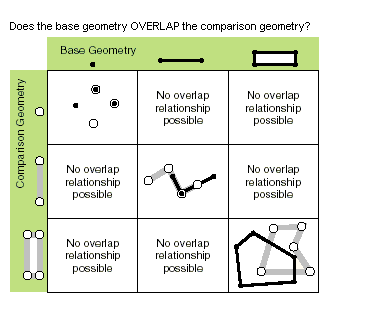pointFromAngleAndDistance (angle, distance, {method}) Returns a point at a given angle in degrees and distance in the units of the geometry's spatial reference using the specified measurement type. projectAs (spatial_reference, {transformation_name}) Projects a geometry and optionally applies a geotransformation. To project, the geometry must have a spatial reference, and not have an unknown coordinate system. The new spatial reference system passed to the method defines the output coordinate system. If either spatial reference is unknown, the coordinates will not be changed. The z- and m-values are not changed by the projectAs method. symmetricDifference (other) Constructs the geometry that is the union of two geometries minus the instersection of those geometries.The two input geometries must be the same shape type.toCoordString (notation) Converts a PointGeometry to the selected coordinate system notation. touches (second_geometry) Indicates if the boundaries of the geometries intersect. Two geometries touch when the intersection of the geometries is not empty, but the intersection of their interiors is empty. For example, a point touches a polyline only if the point is coincident with one of the polyline end points. Only True relationships are shown in this illustration.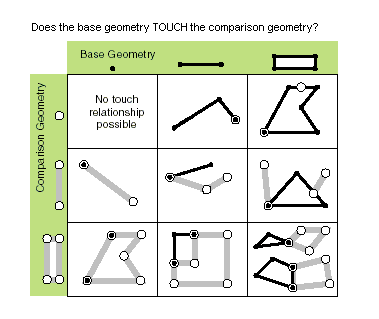union (other) Constructs the geometry that is the set-theoretic union of the input geometries. The two geometries being unioned must be the same shape type.within (second_geometry, {relation}) Indicates if the base geometry is within the comparison geometry. within is the opposite operator of contains. Only True relationships are shown in this illustration.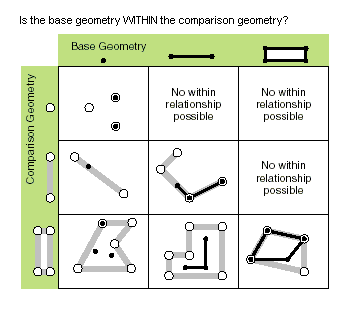The base geometry is within the comparison geometry if the base geometry is the intersection of the geometries and the intersection of their interiors is not empty. within is a Clementini operator, except in the case of an empty base geometry.

Métodos

angleAndDistanceTo (other, {method})
 Parámetro Explicación Tipo de datos other The second geometry. If the geometry is a polygon, the distance is measured to the centroid of the polygon. PointGeometry method The method used to measure distance. GEODESIC—The shortest line between any two points on the earth's surface on a spheroid (ellipsoid). One use for a geodesic line is to determine the shortest distance between two cities for an airplane's flight path. This is also known as a great circle line if based on a sphere rather than an ellipsoid.GREAT_ELLIPTIC—The line on a spheroid (ellipsoid) defined by the intersection at the surface by a plane that passes through the center of the spheroid and the start and endpoints of a segment. This is also known as a great circle when a sphere is used.LOXODROME—The line follows a single compass bearing, or azimuth. Great circle routes are often broken into a series of loxodromes, which simplifies navigation. This is also known as a rhumb line.PLANAR—Planar measurements use 2D Cartesian mathematics to calculate lengths and areas. This option is only available when measuring in a projected coordinate system and the 2D plane of that coordinate system will be used as the basis for the measurements. Planar measurements reflect the projection of geographic data onto a 2D surface, and do not account for the curvature of the earth.PRESERVE_SHAPE—This type calculates the area or length of the geometry on the surface of the earth ellipsoid, for geometry defined in a projected or geographic coordinate system. This option preserves the shape of the geometry in its coordinate system.(El valor predeterminado es GEODESIC) String
Valor de retorno
 Tipo de datos Explicación tuple Returns a tuple of angle (in degrees) and distance (in meters) to another point.
boundary ()
Valor de retorno
 Tipo de datos Explicación Object A polygon's boundary is a polyline. A polyline's boundary is a multipoint, corresponding to the endpoints of the line. A point or multipoint's boundary is an empty point or multipoint.
buffer (distance)
 Parámetro Explicación Tipo de datos distance The buffer distance.The buffer distance is in the same units as the geometry that is being buffered. A negative distance can only be specified against a polygon geometry. Double
Valor de retorno
 Tipo de datos Explicación Polygon The buffered polygon geometry.
clip (envelope)
 Parámetro Explicación Tipo de datos envelope An Extent object used to define the clip extent. Extent
Valor de retorno
 Tipo de datos Explicación Object An output geometry clipped to the specified extent.
contains (second_geometry, {relation})
 Parámetro Explicación Tipo de datos second_geometry A second geometry. Object relation The spatial relationship type.BOUNDARY— Relationship has no restrictions for interiors or boundaries.CLEMENTINI— Interiors of geometries must intersect. Specifying CLEMENTINI is equivalent to specifying None. This is the default.PROPER— Boundaries of geometries must not intersect.(El valor predeterminado es None) String
Valor de retorno
 Tipo de datos Explicación Boolean A return Boolean value of True indicates this geometry contains the second geometry.
convexHull ()
Valor de retorno
 Tipo de datos Explicación Object The resulting geometry. The convex hull of a single point is the point itself.
crosses (second_geometry)
 Parámetro Explicación Tipo de datos second_geometry A second geometry. Object
Valor de retorno
 Tipo de datos Explicación Boolean A return Boolean value of True indicates the two geometries intersect in a geometry of a lesser shape type.
difference (other)
 Parámetro Explicación Tipo de datos other A second geometry. Object
Valor de retorno
 Tipo de datos Explicación Object The resulting geometry.
disjoint (second_geometry)
 Parámetro Explicación Tipo de datos second_geometry A second geometry. Object
Valor de retorno
 Tipo de datos Explicación Boolean A return Boolean value of True indicates that the two geometries share no points in common.
distanceTo (other)
 Parámetro Explicación Tipo de datos other A second geometry. Object
Valor de retorno
 Tipo de datos Explicación Double The distance between the two geometries.
equals (second_geometry)
 Parámetro Explicación Tipo de datos second_geometry A second geometry. Object
Valor de retorno
 Tipo de datos Explicación Boolean A return Boolean value of True indicates that the two geometries are of the same shape type and define the same set of points in the plane.
getGeohash (precision)
 Parámetro Explicación Tipo de datos precision The precision length of the hash string to return for the PointGeometry. The minimum length is 1 and the maximum length is 20. The default length is 8.(El valor predeterminado es 8) Integer
Valor de retorno
 Tipo de datos Explicación String getGeohash returns a geohash string based on the input point geometry latitude and longitude coordinates and the length of precision.

The spatial reference of the point geometry must be in a geographic coordinate system to return an accurate geohash.

import arcpy

# Spatial reference set to GCS_WGS_1984
spatial_reference = arcpy.SpatialReference(4326)
pnt = arcpy.Point(-88.236, 40.096)
pnt_geometry = arcpy.PointGeometry(pnt, spatial_reference)
print(pnt_geometry.getGeohash(6))  # dp1k05
getPart ({index})
 Parámetro Explicación Tipo de datos index The index position of the geometry. Integer
Valor de retorno
 Tipo de datos Explicación Array The resultant Array object.
intersect (other, dimension)
 Parámetro Explicación Tipo de datos other The second geometry. Object dimension The topological dimension (shape type) of the resulting geometry. 1—A zero-dimensional geometry (point or multipoint). 2—A one-dimensional geometry (polyline). 4—A two-dimensional geometry (polygon). Integer
Valor de retorno
 Tipo de datos Explicación Object A new geometry (point, multipoint, polyline, or polygon) that is the geometric intersection of the two input geometries.
overlaps (second_geometry)
 Parámetro Explicación Tipo de datos second_geometry A second geometry. Object
Valor de retorno
 Tipo de datos Explicación Boolean A return Boolean value of True indicates the intersection of the two geometries has the same dimension as one of the input geometries.
pointFromAngleAndDistance (angle, distance, {method})
 Parámetro Explicación Tipo de datos angle The angle in degrees to the returned point. Double distance The distance in the units of the geometry's spatial reference to the returned point. Double method PLANAR measurements reflect the projection of geographic data onto the 2D surface (in other words, they will not take into account the curvature of the earth). GEODESIC, GREAT_ELLIPTIC, LOXODROME, or PRESERVE_SHAPE measurement types can be chosen as an alternative if desired. GEODESIC—The shortest line between any two points on the earth's surface on a spheroid (ellipsoid). One use for a geodesic line is when you want to determine the shortest distance between two cities for an airplane's flight path. This is also known as a great circle line if based on a sphere rather than an ellipsoid.GREAT_ELLIPTIC—The line on a spheroid (ellipsoid) defined by the intersection at the surface by a plane that passes through the center of the spheroid and the start and endpoints of a segment. This is also known as a great circle when a sphere is used.LOXODROME—A loxodrome is not the shortest distance between two points but instead defines the line of constant bearing, or azimuth. Great circle routes are often broken into a series of loxodromes, which simplifies navigation. This is also known as a rhumb line.PLANAR—Planar measurements use 2D Cartesian mathematics to calculate lengths and areas. This option is only available when measuring in a projected coordinate system, and the 2D plane of that coordinate system will be used as the basis for the measurements.PRESERVE_SHAPE—This type calculates the area or length of the geometry on the surface of the earth ellipsoid for geometry defined in a projected or geographic coordinate system. This option preserves the shape of the geometry in its coordinate system.(El valor predeterminado es GEODESIC) String
Valor de retorno
 Tipo de datos Explicación PointGeometry Returns a point at a given angle and distance in degrees and meters.
projectAs (spatial_reference, {transformation_name})
 Parámetro Explicación Tipo de datos spatial_reference The new spatial reference. This can be a SpatialReference object or the coordinate system name. SpatialReference transformation_name The geotransformation name. String
Valor de retorno
 Tipo de datos Explicación Object The projected geometry.
symmetricDifference (other)
 Parámetro Explicación Tipo de datos other A second geometry. Object
Valor de retorno
 Tipo de datos Explicación Object The resulting geometry.
toCoordString (notation)
 Parámetro Explicación Tipo de datos notation The coordinate system notation to be generated. DD— Decimal degrees. For example, 34.05719570N 117.19647020WDDM— Degrees decimal minutes. For example, 34 03.43174200N 117 11.78821200WDDS— Degree Minute Seconds. For example, 34 03 25.90452000N 117 11 47.29272000WGARS— Global Area Reference System. Based on latitude and longitude, it divides and subdivides the world into cells. For example, 126LJ47GEOREF— World Geographic Reference System. For example, EJCE4821203432MGRS— Military Grid Reference System. For example, 11SMT8186968515USNG— United States National Grid. For example, 11S MT 81869 68515UTM— Universal Transverse Mercator. For example, 11S 481868 3768515UTMNS— Universal Transverse Mercator (no spaces). For example, 11N4818683768515 None
Valor de retorno
 Tipo de datos Explicación String The point in the chosen coordinate system notation.
touches (second_geometry)
 Parámetro Explicación Tipo de datos second_geometry A second geometry. Object
Valor de retorno
 Tipo de datos Explicación Boolean A return Boolean value of True indicates the boundaries of the geometries intersect.
union (other)
 Parámetro Explicación Tipo de datos other A second geometry. Object
Valor de retorno
 Tipo de datos Explicación Object The resulting geometry.
within (second_geometry, {relation})
 Parámetro Explicación Tipo de datos second_geometry A second geometry. Object relation The spatial relationship type.BOUNDARY— Relationship has no restrictions for interiors or boundaries.CLEMENTINI— Interiors of geometries must intersect. Specifying CLEMENTINI is equivalent to specifying None. This is the default.PROPER— Boundaries of geometries must not intersect.(El valor predeterminado es None) String
Valor de retorno
 Tipo de datos Explicación Boolean A return Boolean value of True indicates this geometry is contained within the second geometry.

Muestra de código

PointGeometry example

Create a point feature class from scratch.

import arcpy

# A list of coordinate pairs
pointList = [[1, 2], [3, 5], [7, 3]]

# Create an empty Point object
point = arcpy.Point()

# A list to hold the PointGeometry objects
pointGeometryList = []

# For each coordinate pair, populate the Point object and create a new
# PointGeometry object
for pt in pointList:
point.X = pt
point.Y = pt

pointGeometry = arcpy.PointGeometry(point)
pointGeometryList.append(pointGeometry)

# Create a copy of the PointGeometry objects, by using pointGeometryList as
# input to the CopyFeatures tool.
arcpy.CopyFeatures_management(pointGeometryList, "c:/geometry/a.gdb/points")